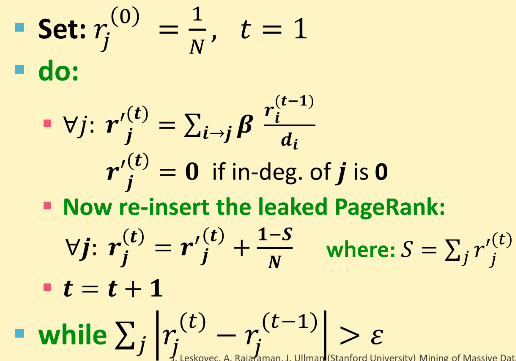NIUHE

Web也可以看作一张图。

Web as a Graph

• Web 可以看作一个有向图
• 节点： 网页
• 边 ： 超链接

Web网络图举例：

PageRank

存在性和唯一性

$r_j^{(t+1)} = _{i j}$ 或者 $r = M r$

• 这个东西（$\vec r$）是否收敛？
• 这个东西是否收敛到我们期待的结果？
• 得到的结果是否有意义？

Problem : Spider Traps

Google解决这个问题的办法是：到达某个节点后 * 有 $\beta$ 的概率随机找一个链接过去 * 剩下 $1-\beta$ 的概率跳到一个随机的页面 * 一般 $\beta$ 的值在 $0.8$$0.9$ 之间

Random Jumps

• 在每一步，漫步者有两个选择：
• $\beta$ 的可能性随机选一个链接
• $1-\beta$ 的可能性跳转到随机页面

PageRank 计算方程 就变成了： > $r_j = _{i j} + (1-)$

The Google Matrix A > $A = M + (1 - ) e e^T$

What is $\beta$ ? 实践表明 $\beta$ 取在 $0.8$~ $0.9$ 之间较好，其中 $\beta=0.85$ 时每隔五步跳转一次。

实际上是如何计算PageRank的？

最终的算法

• 输入 ： 图 $G$ 和 参数 $\beta$
• $G$ 是有向图而且可以有 spider trapsdead ends
• 概率参数 $\beta$
• 输出：Pagerank 向量 $\vec r$